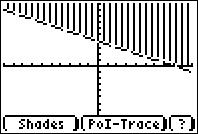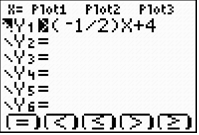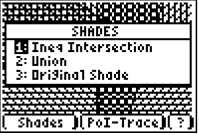••• ##### Device
• TI-83 Plus Family
• TI-84 Plus
• TI-84 Plus Silver Edition
•TI-84 Plus C Silver Edition
•TI-84 Plus CE

# Algebra II: Border Patrol

by Texas Instruments#### Overview

In this activity, students will graph one linear inequality and then graph a system of linear inequalities as they apply to an area of land. Students will practice graphing systems of inequalities and determining if a points lies in the solution. In the extension, students are challenged to write a system of inequalities that describe the area of Mississippi.

#### Key Steps

•Using the Inequality application, students will graph an inequality function that describes the region of the United States on the US/Mexico border.

•Students will graph a system of linear inequalities to determine if a points lies in the solution.

•Students will determine the solution set by examining the graph.